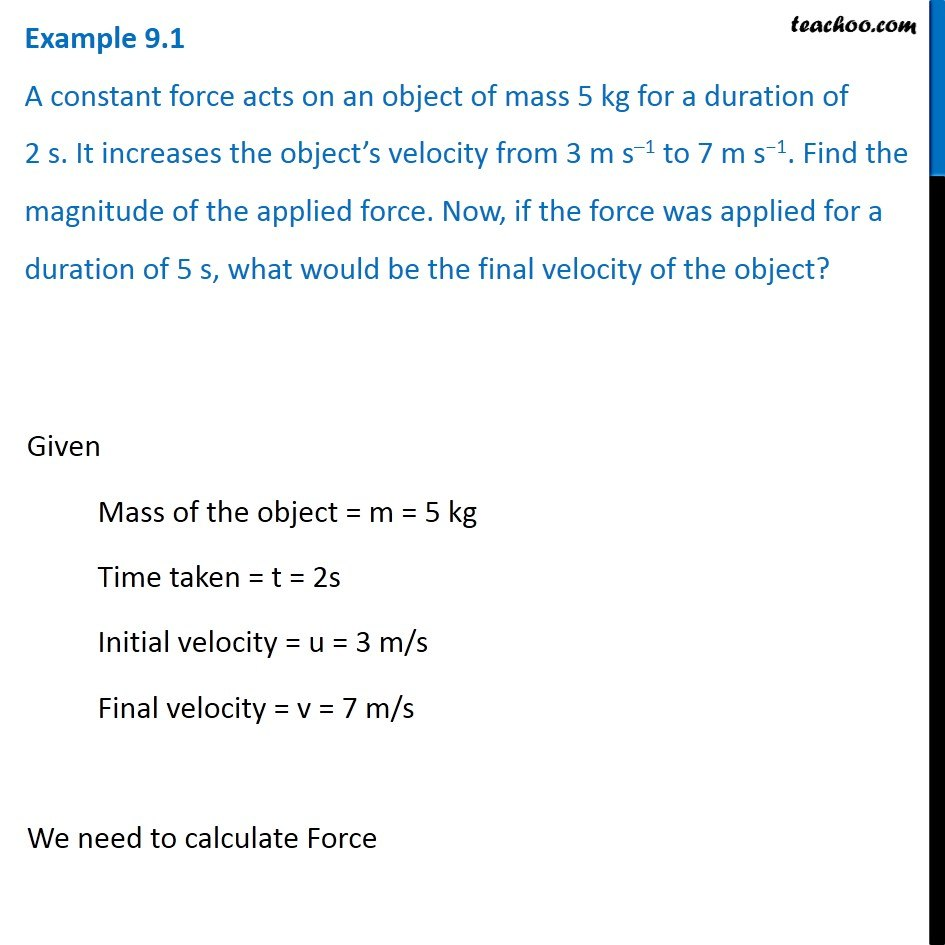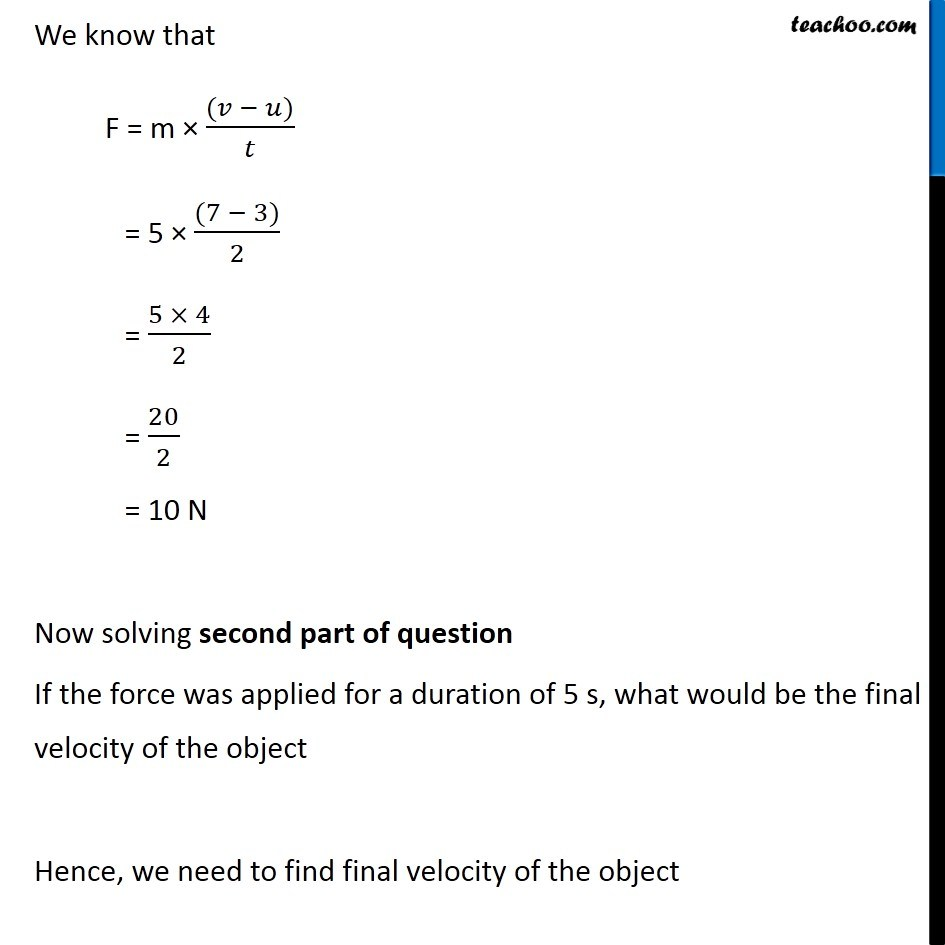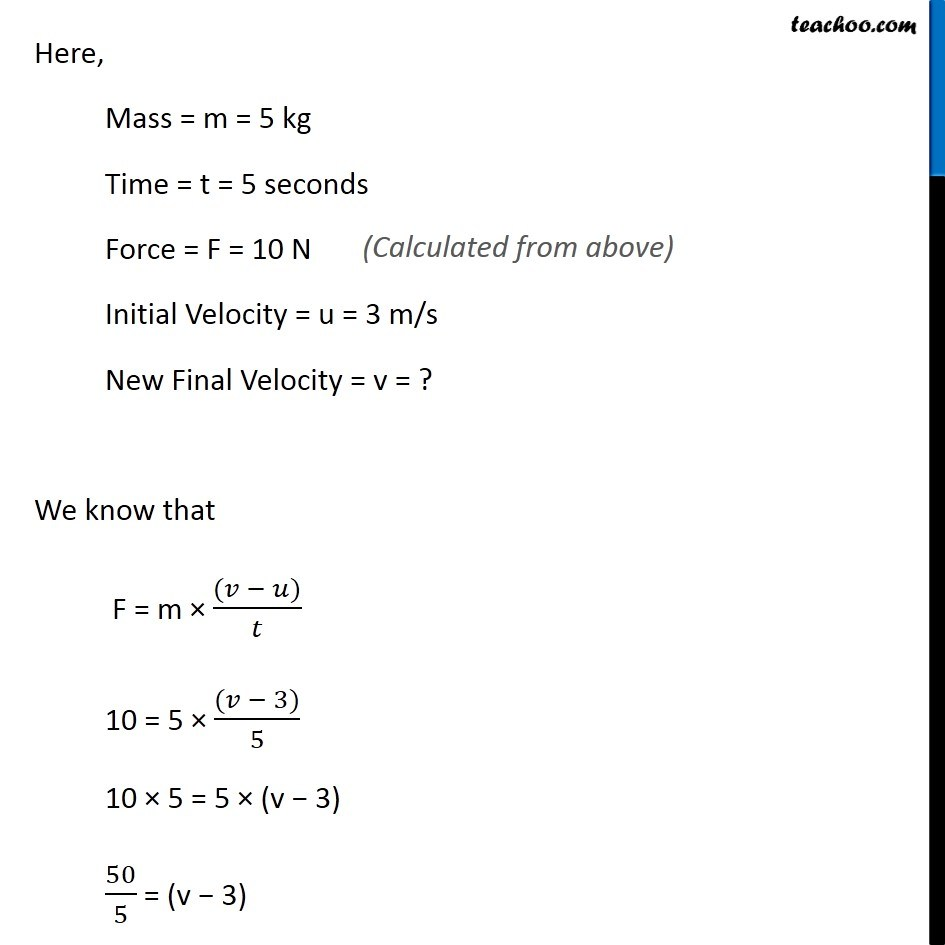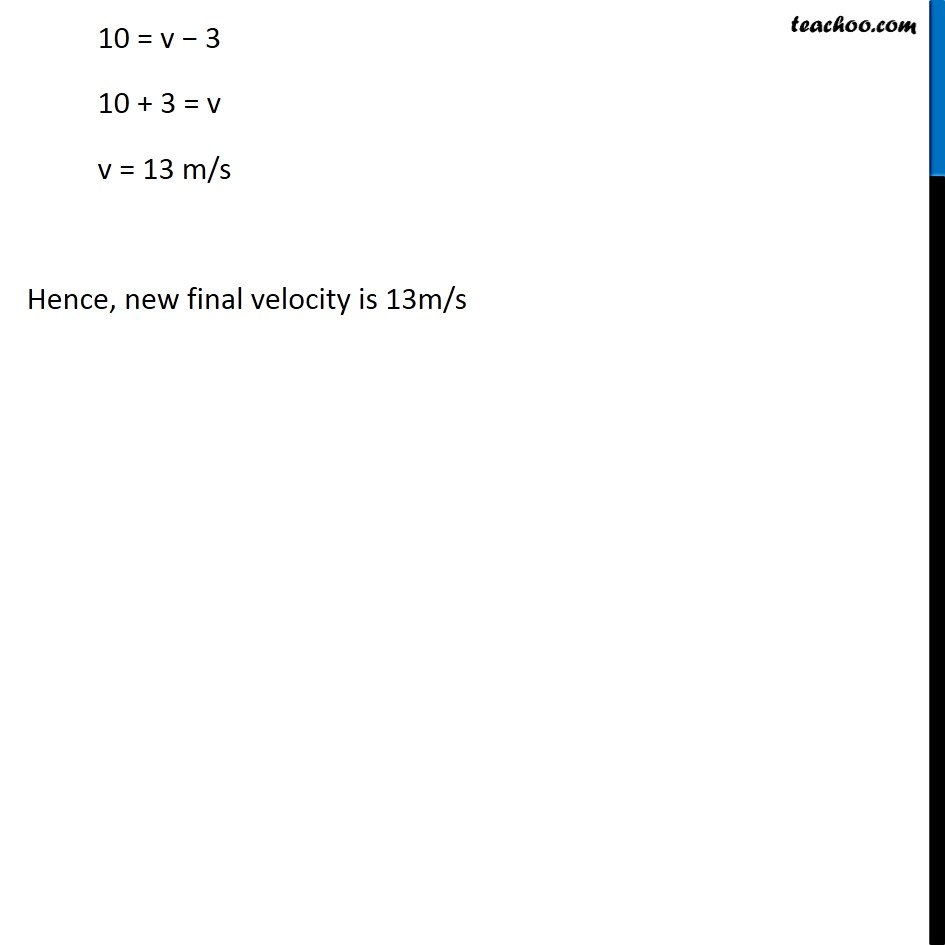Examples from NCERT Book

Class 9
Chapter 9 Class 9 - Force and Laws Of MotionLearn in your speed, with individual attention - Teachoo Maths 1-on-1 Class

### Transcript

Example 9.1 A constant force acts on an object of mass 5 kg for a duration of 2 s. It increases the object’s velocity from 3 m s–1 to 7 m s−1. Find the magnitude of the applied force. Now, if the force was applied for a duration of 5 s, what would be the final velocity of the object? Given Mass of the object = m = 5 kg Time taken = t = 2s Initial velocity = u = 3 m/s Final velocity = v = 7 m/s We need to calculate Force We know that F = m × ((𝑣 − 𝑢))/𝑡 = 5 × ((7 − 3))/2 = (5 × 4)/2 = 20/2 = 10 N Now solving second part of question If the force was applied for a duration of 5 s, what would be the final velocity of the object Hence, we need to find final velocity of the object Here, Mass = m = 5 kg Time = t = 5 seconds Force = F = 10 N Initial Velocity = u = 3 m/s New Final Velocity = v = ? We know that F = m × ((𝑣 − 𝑢))/𝑡 10 = 5 × ((𝑣 − 3))/5 10 × 5 = 5 × (v − 3) 50/5 = (v − 3) 10 = v − 3 10 + 3 = v v = 13 m/s Hence, new final velocity is 13m/s